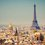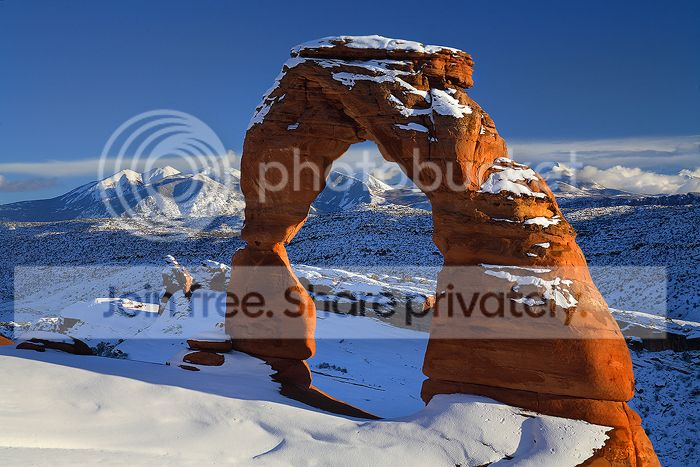# Can We Transform The World Into a Doughnut?

In the mathematical area called Topology. A sphere (a geometric solid like a ball) is homologous or similar to a Torus (another geometric 3 dimensional solid shape like the shape of a ring doughnut).

Obviously, there is a difference between the shape of the ball and a doughnut. A ball is perfectly round, while the doughnut has a hole in the middle like the floaters we use when we swim. But in topology, they say that "There's not much a difference."

Another one. In topology, a coffee cup is the same shape as the doughnut. The explanation they give is: Because they both have a hole. The coffee cup's hole is the space between the handle. And then, just do some transformation etc...etc... and there you have it, your mug turned into a doughnut!

By using the logic of math if a=b, and b=c, then a=c, substituting the ball for a, doughnut for b and mug for c, then , that implies, that the ball is the same as the coffee cup! Okay, then.

Now, isn't it that basically, the world is the shape of a sphere, or a ball? I mean. Yes, I know that it is not perfectly round. But it has no hole or puncture whatsoever anywhere as the doughnut, a mug, or a floater has, right? But again, topology has something to say: If we can transform the ball into a doughnut and a coffee cup, we can transform the world into a doughnut.

Imagine that!

The world can be transformed into a doughnut (sphere becoming a torus, to be more generic) by the mathematics of what topologists now call: Surgery, invented by Mr. G. Perelman.

Topology is really awesome, and I adore topologists alot. And before I close this, I just want to add the quote of the Fields Medal recipient on 2007 (but declined to), Mathematician and topologist Grigory Perelman: "I do not need a prize,cash, or even fame. I could shrink the world into a single point, what more can I ask for?"Note by Les Narvasa
6 years, 7 months ago

This discussion board is a place to discuss our Daily Challenges and the math and science related to those challenges. Explanations are more than just a solution — they should explain the steps and thinking strategies that you used to obtain the solution. Comments should further the discussion of math and science.

When posting on Brilliant:

• Use the emojis to react to an explanation, whether you're congratulating a job well done , or just really confused .
• Ask specific questions about the challenge or the steps in somebody's explanation. Well-posed questions can add a lot to the discussion, but posting "I don't understand!" doesn't help anyone.
• Try to contribute something new to the discussion, whether it is an extension, generalization or other idea related to the challenge.
• Stay on topic — we're all here to learn more about math and science, not to hear about your favorite get-rich-quick scheme or current world events.

MarkdownAppears as
*italics* or _italics_ italics
**bold** or __bold__ bold
- bulleted- list
• bulleted
• list
1. numbered2. list
1. numbered
2. list
Note: you must add a full line of space before and after lists for them to show up correctly
paragraph 1paragraph 2

paragraph 1

paragraph 2

[example link](https://brilliant.org)example link
> This is a quote
This is a quote
    # I indented these lines
# 4 spaces, and now they show
# up as a code block.

print "hello world"
# I indented these lines
# 4 spaces, and now they show
# up as a code block.

print "hello world"
MathAppears as
Remember to wrap math in $$ ... $$ or $ ... $ to ensure proper formatting.
2 \times 3 $2 \times 3$
2^{34} $2^{34}$
a_{i-1} $a_{i-1}$
\frac{2}{3} $\frac{2}{3}$
\sqrt{2} $\sqrt{2}$
\sum_{i=1}^3 $\sum_{i=1}^3$
\sin \theta $\sin \theta$
\boxed{123} $\boxed{123}$

## Comments

Sort by:

Top Newest

Do not forget graph theory too.

Euler’s Formula

Remember Leonard Euler, the mathematician who invented Graph Theory? He played around with many different graphs and discovered another interesting property related to the number of vertices, edges and faces. This applies to planar graphs, graphs which can be drawn so that no edges overlap unless they meet at a vertexIf we have a look at the last two rows of the table above you will notice that “faces + vertices” is always one more than the number of edges, or that

faces + vertices = edges + 1

Euler managed to prove that this is true for all planar graphs, and it is known as Euler’s formula.

Four Colour Theorem

Here is another famous problem that is related to Graph Theory:

We want to colour a map of countries so that no two adjacent countries get the same colour. What is the maximum amount of colours we need to be able to colour any map?

Here is an example that works with four colours. Notice that it wouldn’t work with only three colours: eventually we would always arrive at a point where we cannot colour a state because all three colours have already been used on its neighbours.But are four colours always enough? Or are there certain maps for which we need at least five colours? In 1852, Francis Guthrie together with Augustus De Morgan (1806 – 1871) conjectured that four colours are enough to colour any map: the four colour theorem.

And while the problem is easy to understand, proving it seemed to be rather more difficult. There were several early proofs, and in some cases it took more than 10 years until a mistake was found. It is reasonably simple to prove that five colours are always enough – but the four colour problem remained unsolved for many years.

The four colour theorem can be translated into graph theory: we convert every country into a vertex and whenever there is a border between two countries we connect their vertices with an edge. Now we want to colour a graph so that vertices connected by an edge get different colours.- 6 years, 7 months ago

Log in to reply

There's all kinds of natural arches in the world, so we don't need to do a transform to change the genus of the Earth. It's already a doughnut.Natural Arch

- 6 years, 7 months ago

Log in to reply

But a doughnut only has 1 hole. The existence of many natural arches would mean that we are a many-holed doughnut!

So, maybe a waffle fry?image

Staff - 6 years, 7 months ago

Log in to reply

You know, this reminds me of the Jewel Cave complex in the Black Hills of South Dakota. Yeah, that would be a better description. It has over 170 miles of passages that look a lot like your picture. So, what's the topological genus of Planet Earth? Some really big number.

Topology is the study of geometrical properties that are unaffected by certain kinds of continuous transformations, which is similar to the study of invariant properties in physics and symmetry defined by transformations. The classic continuous transformation in topology that we're familiar with preserves the genus of the objection, i.e., a sphere would stay a sphere and is not transformable into a doughnut. However, topology studies many other kinds of continuous transformations that don't necessarily preserve genus.

- 6 years, 7 months ago

Log in to reply

:D it made me laugh. yeah, I got the Idea.

- 6 years, 6 months ago

Log in to reply

×

Problem Loading...

Note Loading...

Set Loading...